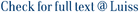Given a weighted directed graph G = (V, A), the minimum feedback arc set problem consists of finding a minimum weight set of arcs A' subset of or equal to A such that the directed graph (V, A A') is acyclic. Similarly, the minimum feedback vertex set problem consists of finding a minimum weight set of vertices containing at least one vertex for each directed cycle. Both problems are NP-complete. We present simple combinatorial algorithms for these problems that achieve an approximation ratio bounded by the length, in terms of number of arcs, of a longest simple cycle of the digraph. (C) 2003 Elsevier Science B.V. All rights reserved.

Combinatorial algorithms for feedback problems in directed graphs / Demetrescu, Camil; Finocchi, Irene. - In: INFORMATION PROCESSING LETTERS. - ISSN 0020-0190. - 86:3(2003), pp. 129-136. [10.1016/s0020-0190(02)00491-x]

### Combinatorial algorithms for feedback problems in directed graphs

#### Abstract

Given a weighted directed graph G = (V, A), the minimum feedback arc set problem consists of finding a minimum weight set of arcs A' subset of or equal to A such that the directed graph (V, A A') is acyclic. Similarly, the minimum feedback vertex set problem consists of finding a minimum weight set of vertices containing at least one vertex for each directed cycle. Both problems are NP-complete. We present simple combinatorial algorithms for these problems that achieve an approximation ratio bounded by the length, in terms of number of arcs, of a longest simple cycle of the digraph. (C) 2003 Elsevier Science B.V. All rights reserved.
##### Scheda breve Scheda completa Scheda completa (DC)approximation algorithms; combinatorial optimization; directed graphs; feedback problems
File in questo prodotto:
Non ci sono file associati a questo prodotto.
##### Pubblicazioni consigliate

Caricamento pubblicazioni consigliate

I documenti in IRIS sono protetti da copyright e tutti i diritti sono riservati, salvo diversa indicazione.

Utilizza questo identificativo per citare o creare un link a questo documento: `http://hdl.handle.net/11385/192649`
##### Citazioni
•37
•26